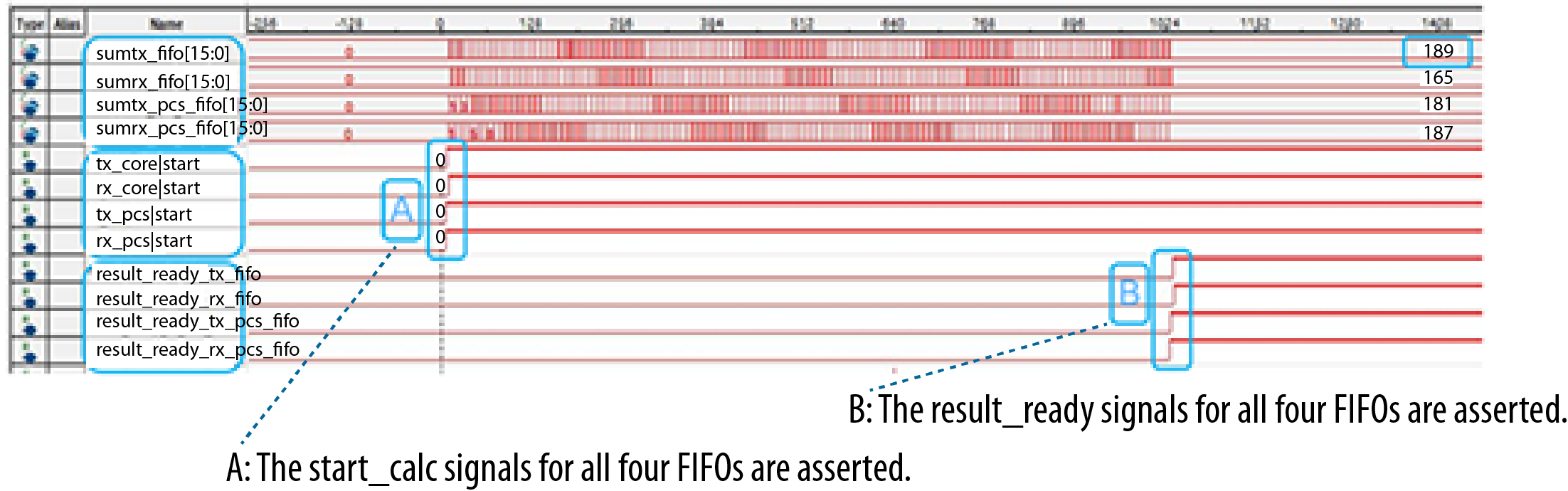ID 683621
Date 10/05/2023
Public

## 2.4.3.1.2. FIFO Latency Calculation

Latency through FIFOs (in PC mode) may vary from reset to reset. You can determine that variation by using the appropriate FIFO latency calculation logic. The phase measuring technique measures the distance between read and write counters. The phase measuring circuit is designed to work in the case of a phase comp FIFO and in the case of a phase comp FIFO where the read and write pointers may have an exact 2:1 ratio.

To measure the fullness of the FIFO, run your sampling clock at a rate that is not equal to the parallel clock. The guideline for calculating the sampling clock is that the sampling clock sweeps various phase relationships relative to the parallel clock. Using a simple counter, you should determine how often the resulting pulse is a 1 versus a 0. You can find this implementation in the FIFO latency calculation logic referenced below.

Figure 85. Phase Measuring FIFO

Below is an example implementation of the FIFO latency calculation logic. Each FIFO in phase compensation mode has a latency measurement port (read only) available at the PCS-Core interface. Each latency measurement port supplies the latency pulse whose duty cycle depends on the FIFO latency. This latency pulse is further processed in the FPGA fabric to calculate FIFO latency. There are four similar pulses for the four FIFOs, and you must perform latency calculations on all of them separately. The IP represented in the following figure represents the logic in the FPGA fabric that performs the latency calculation. This IP is not a part of the Native PHY IP core, so you must instantiate it exclusively. Output of the IP is the Total FIFO count bus. This bus produces the result of the FIFO latency calculation. This result can only be read when the calculation is complete, and is denoted by the result_ready signal coming out of the IP. Refer to FIFO Latency Calculation.

Figure 86. FIFO Latency Calculation

Once result_ready is asserted, you can read data on the Total FIFO count bus and calculate latency time using the following equation.

Figure 87. FIFO Latency Formula

The latency pulse available at a latency measurement port of a FIFO appears as shown below. The duty cycle of the pulse depends on the difference between the read and write pointers at a particular instant. The duty cycle of a pulse varies with time as both read and write pointers move. To find the actual latency through the FIFO, the IP block calculates the average of latency pulse duty cycles for an appropriate duration.

As seen in the figure below, a sampling clock (LATENCY_SCLK) samples the pulse. After the sampling duration, all the samples of 1s are accumulated and appear at the Total FIFO count output bus, which is then used in FIFO Latency Calculation to calculate latency (in nsec). The Number of pulses parameter is the actual number of latency pulses for which the latency calculation logic executes. This parameter defines the execution time of the latency calculation logic. The design file referenced below defaults to 128 latency pulses to calculate FIFO latency. Due to hardware limitations, the sampling clock cannot be higher than 260 MHz.

Figure 88. FIFO Latency Measurement Port Number of pulses and the LATENCY_SCLK period vary depending on your particular implementation.
Figure 89. FIFO Latency Calculation Logic
Table 91.  FIFO Latency Calculation IP Signals
Symbol Input/Output Description
latency_sclk Input Sampling clock required by the latency calculation logic
start_calc Input Control signal to start the latency calculation
fifo_latency_pulse Input Latency pulse
total_fifo_count Output Result from the latency calculation logic
result_ready Output Status signal that signifies completion of the latency calculation logic
Figure 90. FIFO Latency Calculation Logic for Four FIFOsPoint A is the assertion of start_calc signals, and point B is an assertion of result_ready signals. The total_fifo_count signal is valid after result_ready is asserted.Figure 91. FIFO Latency Formula for all FIFOs

Follow these guidelines for the preferred frequency for latency_sclk:

• latency_sclk must be ≤ 260 MHz.
• The ratio of parallel clock to latency_sclk, when expressed in lowest terms, should have denominator of at least 50, but preferably, more than 100. The ideal resultant latency_sclk should be between 200 MHz to 260 MHz.
• Do not use a latency_sclk frequency that is a multiple of tx_clkout.
• Examples:
Table 92.  Parallel Clock to latency_sclk Ratio Scenarios
tx_clkout Frequency Correct latency_sclk Frequency Incorrect latency_sclk Frequency
150 MHz 209 MHz 160 MHz
245 MHz 247 MHz 250 MHz
300 MHz 247 MHz 260 MHz

You can create latency_sclk using the IOPLL or the external reference clock.